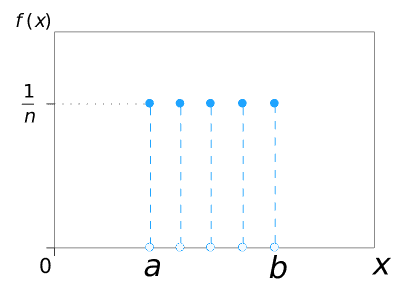# Probability and Statistics Symbols

A comprehensive collection of the most common symbols in probability and statistics, categorized by function into charts and tables along with each symbol's term, meaning and example.

Probability and statistics correspond to the mathematical study of chance and data, respectively. The following reference list documents some of the most notable symbols in these two topics, along with each symbol’s usage and meaning.

For readability purpose, these symbols are categorized by function into tables. Other comprehensive lists of math symbols — as categorized by subject and type — can be also found in the relevant pages below (or in the navigational panel).

Get the master summary of mathematical symbols in eBook form — along with each symbol’s usage and LaTeX code.

## Variables

Probability and statistics both employ a wide range of Greek/Latin-based symbols as placeholders for varying objects and quantities. The following table documents the most common of these — along with each symbol’s usage and meaning.

## Operators

In probability and statistics, operators denote mathematical operations which are used to better make sense of data and chances. These include key combinatorial operators, probability-related operators/functions, probability distributions and statistical operators.

### Probability-related Operators

The following are some of the most notable operators related to probability and random variables. For a review on sets, see set operators.

### Probability-distribution-related Operators

#### Discrete Probability Distributions

The following graphs illustrate the probability mass functions of 6 of the key distributions mentioned above.$U\{a, b\}$

## Relational Symbols

Relational symbols are symbols used to denote mathematical relations, which express some connection between two or more mathematical objects or entities. The following table documents the most notable of these in the context of probability and statistics  — along with each symbol’s usage and meaning.

## Notational Symbols

Notational symbols are often conventions or acronyms that don’t fall into the categories of constants, variables, operators and relational symbols. The following table documents some of the most common notational symbols in probability and statistics — along with their respective usage and meaning.

For the master list of symbols, see mathematical symbols. For lists of symbols categorized by subject and type, refer to the relevant pages below for more.

1.